### Basic Geometrical Ideas-Solutions Ex-4.1

CBSE Class –VI Mathematics
NCERT Solutions
Chapter 4
Basic Geometrical Ideas (Ex. 4.1)

Question 1. Use the figure to name: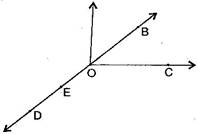(a) Five points
(b) A line
(c) Four rays
(d) Five line segments
Answer:  (a) Five points are: O, B, C, D, E
(b) A line: $\overline{\text{DE}},$$\overline{\text{DB}},$$\overline{\text{OE}},$$\overline{\text{OB}}$
(c) Four rays: $\stackrel{\to }{\text{OD}},$$\stackrel{\to }{\text{OE}},$$\stackrel{\to }{\text{OC}},$$\stackrel{\to }{\text{OB}}$
(d) Four line segments: $\overline{\text{DE}},$$\overline{\text{OE}},$$\overline{\text{OC}},$$\overline{\text{OB}},$$\overline{\text{OD}}$
Question 2. Name the line given in all possible (twelve) ways, choosing only two letters at a time from the four given.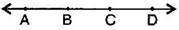Answer: $\overline{\text{AB}},$$\overline{\text{AC}},$$\overline{\text{AD}},$$\overline{\text{BC}},$$\overline{\text{BD}},$$\overline{\text{CD}},$$\overline{\text{BA}},$$\overline{\text{CA}},$$\overline{\text{DA}},$$\overline{\text{CB}},$$\overline{\text{DB}},$$\overline{\text{DC}}$
Question 3. Use the figure to name: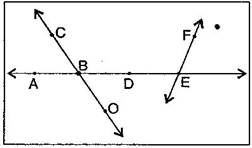(a) Line containing point E.
(b) Line passing through A.
(c) Line on which O lies.
(d) Two pairs of intersecting lines.
Answer: (a) A line containing E = $\overline{\text{AE}}$ or $\overline{\text{FE}}$
(b) A line passing through A = $\overline{\text{AE}}$ or $\overline{\text{DE}}$
(c) A line on which O lies = $\overline{\text{CO}}$ or $\overline{\text{OC}}$
(d) Two pairs of intersecting lines are : $\overline{\text{AD}},$$\overline{\text{CO}}$ and $\overline{\text{AE}}$$\overline{\text{FE}}$
Question 4. How many lines can pass though:
(a) one given point?
(b) two given points
Answer: (a) Infinite number of lines can pass through one given point.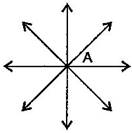(b) Only one line can pass through two given points.
Question 5. Draw a rough figure and label suitably in each of the following cases:
(a) Point P lies on $\overline{\text{AB}}.$
(b) $\overline{\text{XY}}$ and $\overline{\text{PQ}}$ intersect at M.
(c) Line $l$ contains E and F but not D.
(d) $\overline{\text{OP}}$ and $\overline{\text{OQ}}$ meet at O.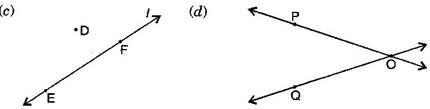Question 6. Consider the following figure of line $\overline{\text{MN}}$. Say whether following statements are true or false in the context of the given figure: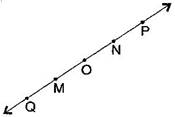(a) Q, M, O, N, P are points on the line $\overline{\text{MN}}$.
(b) M, O, N are points on a line segment $\overline{\text{MN}}$.
(c) M and N are end points of line segment $\overline{\text{MN}}$.
(d) O and N are end points of line segment $\overline{\text{OP}}$.
(e) M is one of the end points of line segment $\overline{\text{QO}}$.
(f) M is point on ray $\stackrel{\to }{\text{OP}}$.
(g) Ray $\stackrel{\to }{\text{OP}}$ is different from ray $\stackrel{\to }{\text{OP}}$.
(h) Ray $\stackrel{\to }{\text{OP}}$same as ray $\stackrel{\to }{\text{OM}}.$.
(i) Ray $\stackrel{\to }{\text{OM}}.$ is not opposite to ray $\stackrel{\to }{\text{OP}}$.
(j) O is not an initial point of $\overline{\text{NP}}$ and $\overline{\text{NM}}$.
Answer: (a) True, (b) True, (c) True, (d) False, (e) False, (f) False, (g) True, (h) False, (i) False, (j) False, (k) True